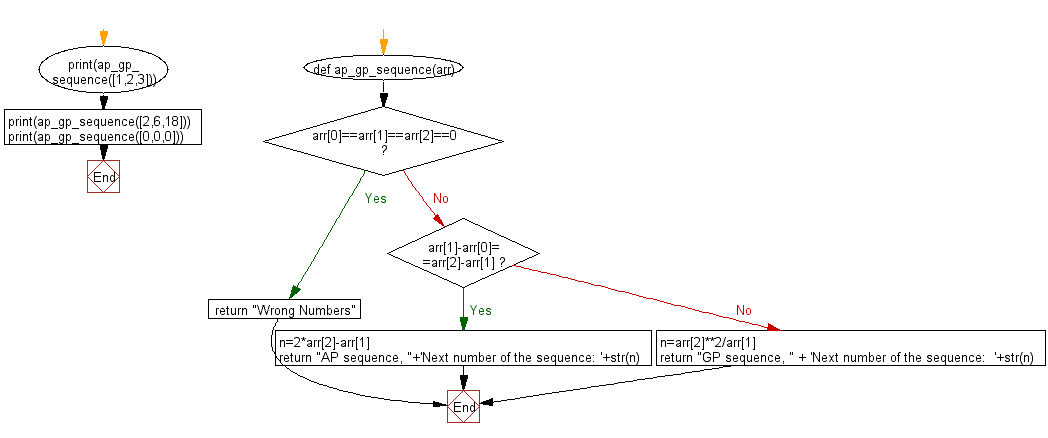﻿ Python: Find the type of the progression and the next successive member of a given three successive members of a sequence - w3resource# Python: Find the type of the progression and the next successive member of a given three successive members of a sequence

## Python Basic - 1: Exercise-27 with Solution

Write a Python program to find the type of the progression (arithmetic progression/geometric progression) and the next successive member of a given three successive members of a sequence.

According to Wikipedia, an arithmetic progression (AP) is a sequence of numbers such that the difference of any two successive members of the sequence is a constant. For instance, the sequence 3, 5, 7, 9, 11, 13, . . . is an arithmetic progression with common difference 2. For this problem, we will limit ourselves to arithmetic progression whose common difference is a non-zero integer. On the other hand, a geometric progression (GP) is a sequence of numbers where each term after the first is found by multiplying the previous one by a fixed non-zero number called the common ratio. For example, the sequence 2, 6, 18, 54, . . . is a geometric progression with common ratio 3. For this problem, we will limit ourselves to geometric progression whose common ratio is a non-zero integer.

Sample Solution:

Python Code:

``````def ap_gp_sequence(arr):
if arr==arr==arr==0:
return "Wrong Numbers"
else:
if arr-arr==arr-arr:
n=2*arr-arr
return "AP sequence, "+'Next number of the sequence: '+str(n)
else:
n=arr**2/arr
return "GP sequence, " + 'Next number of the sequence:  '+str(n)

print(ap_gp_sequence([1,2,3]))
print(ap_gp_sequence([2,6,18]))
print(ap_gp_sequence([0,0,0]))
``````

Sample Output:

```AP sequence, Next number of the sequence: 4
GP sequence, Next number of the sequence:  54.0
Wrong Numbers
```

Pictorial Presentation:Flowchart:## Visualize Python code execution:

The following tool visualize what the computer is doing step-by-step as it executes the said program:

Python Code Editor:

Have another way to solve this solution? Contribute your code (and comments) through Disqus.

What is the difficulty level of this exercise?

Test your Programming skills with w3resource's quiz.

﻿

## Python: Tips of the Day

What is the difference between Python's list methods append and extend?

append: Appends object at the end.

```x = [1, 2, 3]
x.append([4, 5])
print (x)
```

Output:

```[1, 2, 3, [4, 5]]
```

extend: Extends list by appending elements from the iterable.

```x = [1, 2, 3]
x.extend([4, 5])
print (x)
```

Output:

```[1, 2, 3, 4, 5]
```

Ref: https://bit.ly/2AZ6ZFq